# Class 10 Maths Worksheet for Areas Related to Circles

In this page we have Class 10 Maths Worksheet for Areas Related to Circles . Hope you like them and do not forget to like , social share and comment at the end of the page.

1) In a circle of radius 6cm, a chord of length 10cm makes an angle of 110o at the centre of the circle. Find
a)the circumference of the circle
b)the area of the circle
c)the length of the arc AB
d)the area of sector OAB
2) In figure, shows a sector of a circle, centre O, containing an angle θ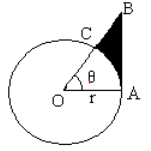Prove that
Perimeter of shaded region is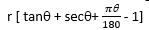Area of shaded region is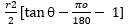3) Find the number of revolutions made by a circular wheel of area 1.54 m2 in rolling a distance of 176 m.
4) The diameters of front and rear wheels of a tractor are 80 cm and 2 m respectively. Find the number of revolutions that rear wheel will make in covering a distance in which the front wheel makes 1400 revolutions
5) A chord 10cm long is drawn in a circle whose radius is 5√2 cm. Find area of both the segments. (Take π=3.14)
6) A chord AB of a circle, of radius 14cm makes an angle of 60o at the centre of the circle. Find the area of the minor segment of the circle. (Use π=22/7)
7) AB is the diameter of a circle, centre O. C is a point ion the circumference such that <COB = θ. The area of the minor segment cut off by AC is equal to twice the area of the sector BOC. Prove that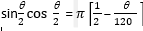8) A chord of a circle subtends an angle of θ at the centre of the circle. The area of the minor segment cut off by the chord is one eighth of the area of the circle. Prove that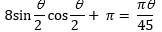9) PQRS is a diameter of a circle of radius 6cm. The lengths PQ, QR and RS are equal. Semi- circles are drawn on PQ and QS as diameters as shown in figure. Find the perimeter and area of shaded region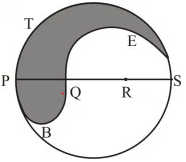10) In an equilateral triangle of side 24cm, a circle is inscribed touching its sides. Find the area of the remaining portion of the triangle. ( Take √3=1.732)
11)A cow is tied with a rope of length 14 m at the corner of a rectangular field of dimensions 20m × 16m. Find the area of the field in which the cow can graze.
12) Four equal circles, each of radius a, touch each other. Show that the area between them is 6a2 /7   (Take π=22/7)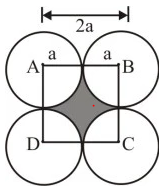13) A rectangular park is 100m by 50m. It is surrounded by semi- circular flower beds all round. Find the cost of leveling the semi- circular flower bed at 60 paise per square metre ( Use π=3.14)
14) Prove that the area of a circular path of uniform width h surrounding a circular region of radius r is πh (2r + h).
15) The inside perimeter of a running track is 400m. The length of each of the straight portion is 90m and the ends are semi- circles. If the track is everywhere 14m wide, find the area of the track. Also find the length of the outer running track.
Answer
1) i) 37.68 cm
(ii) 113.1 cm2
(iii) 11.51 cm2
(iv) 34.5 cm2
5) 14.25 cm2
6) 17.80cm2
9) 12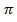cm, 37.71cm2
10) 98.55 cm2

link to this page by copying the following text
Reference Books for class 10

Given below are the links of some of the reference books for class 10 math.

You can use above books for extra knowledge and practicing different questions.

### Practice Question

Question 1 What is $1 - \sqrt {3}$ ?
A) Non terminating repeating
B) Non terminating non repeating
C) Terminating
D) None of the above
Question 2 The volume of the largest right circular cone that can be cut out from a cube of edge 4.2 cm is?
A) 19.4 cm3
B) 12 cm3
C) 78.6 cm3
D) 58.2 cm3
Question 3 The sum of the first three terms of an AP is 33. If the product of the first and the third term exceeds the second term by 29, the AP is ?
A) 2 ,21,11
B) 1,10,19
C) -1 ,8,17
D) 2 ,11,20

Note to our visitors :-

Thanks for visiting our website.
DISCLOSURE: THIS PAGE MAY CONTAIN AFFILIATE LINKS, MEANING I GET A COMMISSION IF YOU DECIDE TO MAKE A PURCHASE THROUGH MY LINKS, AT NO COST TO YOU. PLEASE READ MY DISCLOSURE FOR MORE INFO.